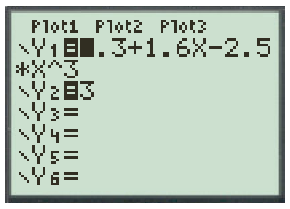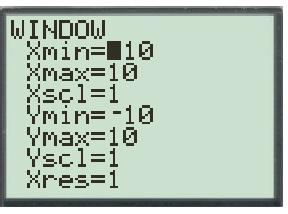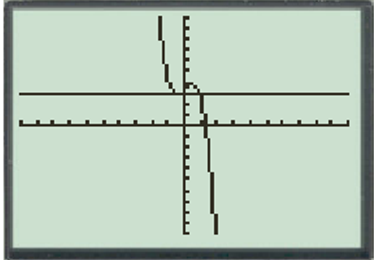# By using T1-83 calculator, determine whether the given function p ( x ) = 3.3 + 1.6 x − 2.5 x 3 is one −to − one.### Precalculus: Mathematics for Calcu...

6th Edition
Stewart + 5 others
Publisher: Cengage Learning
ISBN: 9780840068071### Precalculus: Mathematics for Calcu...

6th Edition
Stewart + 5 others
Publisher: Cengage Learning
ISBN: 9780840068071

#### Solutions

Chapter 2, Problem 85RE
To determine

## By using T1-83 calculator, determine whether the given function p(x)=3.3+1.6x−2.5x3 is one −to − one.

Expert Solution

Hence, p is not one −to − one.

### Explanation of Solution

Given information: Consider the function p(x)=3.3+1.6x2.5x3

Calculation:

Press the Y= Button and enter the function Y1=3.3+1.6×2.5×3 and Y2=3Once this is done on the WINDOW screen

X min=10

X max=10

Y min=10

Y max=10

This gives a range that is good for many

Varied graph types

Set the windowThen click the GRAPH button.From the above graph, observe that the horizontal line that intersects the graph of p more than once.

Hence the horizontal line test p is not one −to − one.

### Have a homework question?

Subscribe to bartleby learn! Ask subject matter experts 30 homework questions each month. Plus, you’ll have access to millions of step-by-step textbook answers!